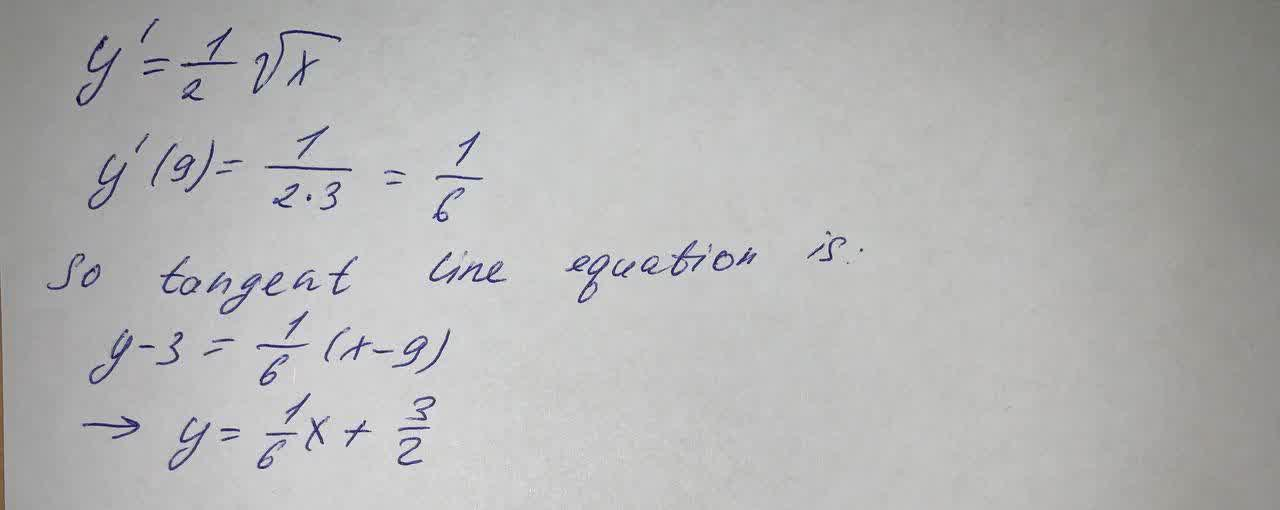# Find an equation of the tangent line to the curve at the given point (9, 3) y=\fracdefazajx 2021-09-19 Answered
Find an equation of the tangent line to the curve at the given point (9, 3)
$$\displaystyle{y}={\frac{{{1}}}{{\sqrt{{{x}}}}}}$$

• Questions are typically answered in as fast as 30 minutes

### Plainmath recommends

• Get a detailed answer even on the hardest topics.
• Ask an expert for a step-by-step guidance to learn to do it yourself.Alara Mccarthy
The solution is written below